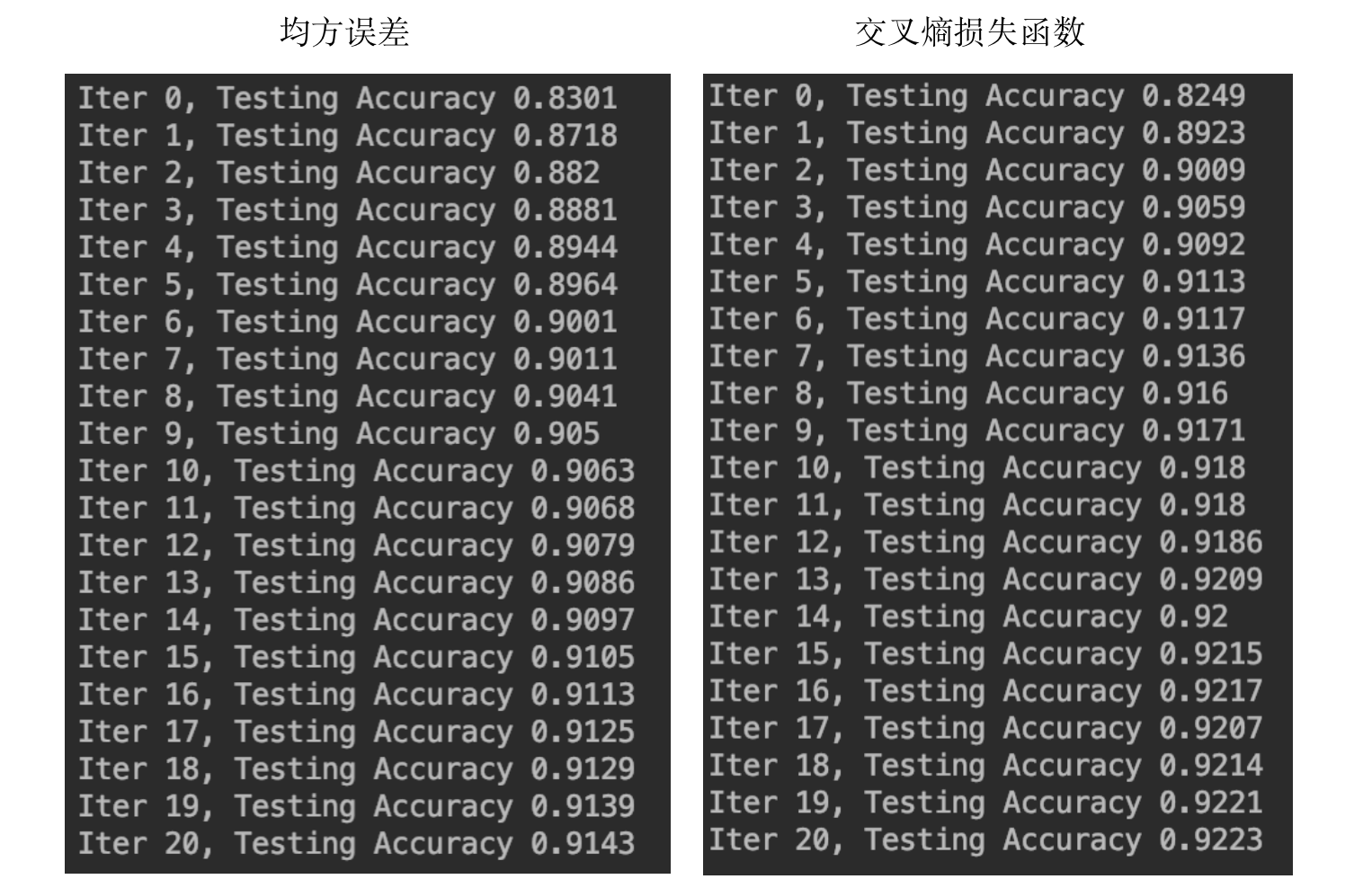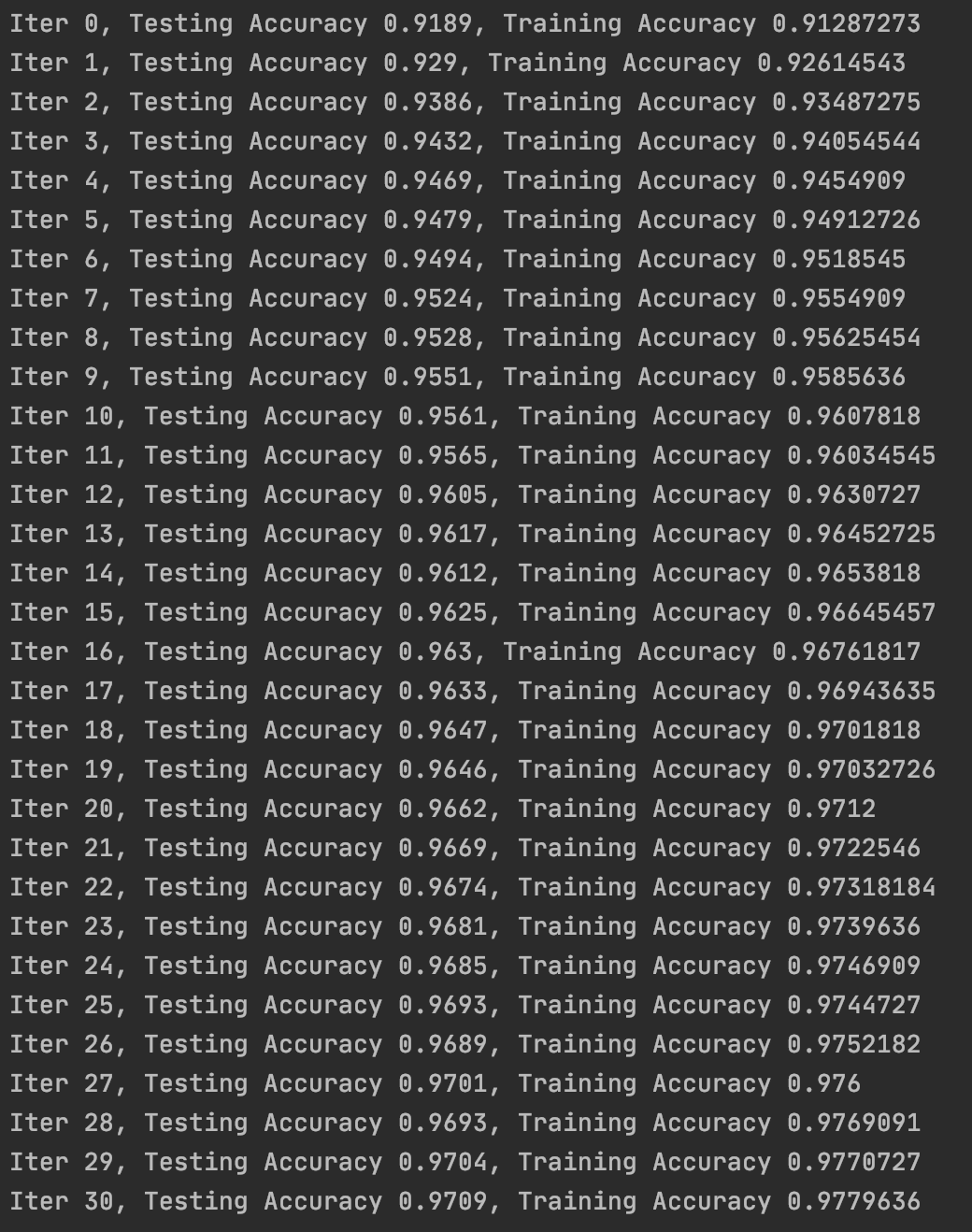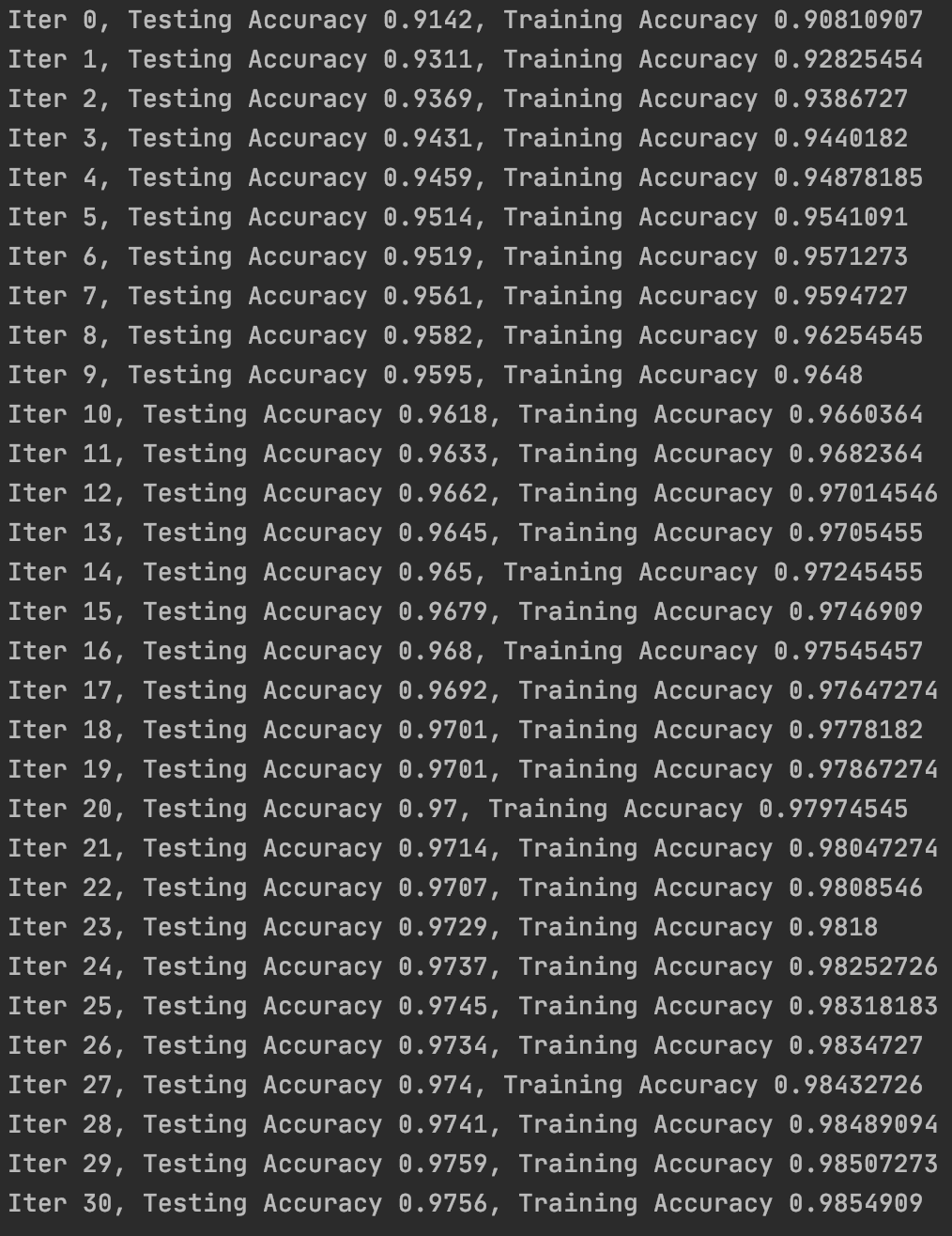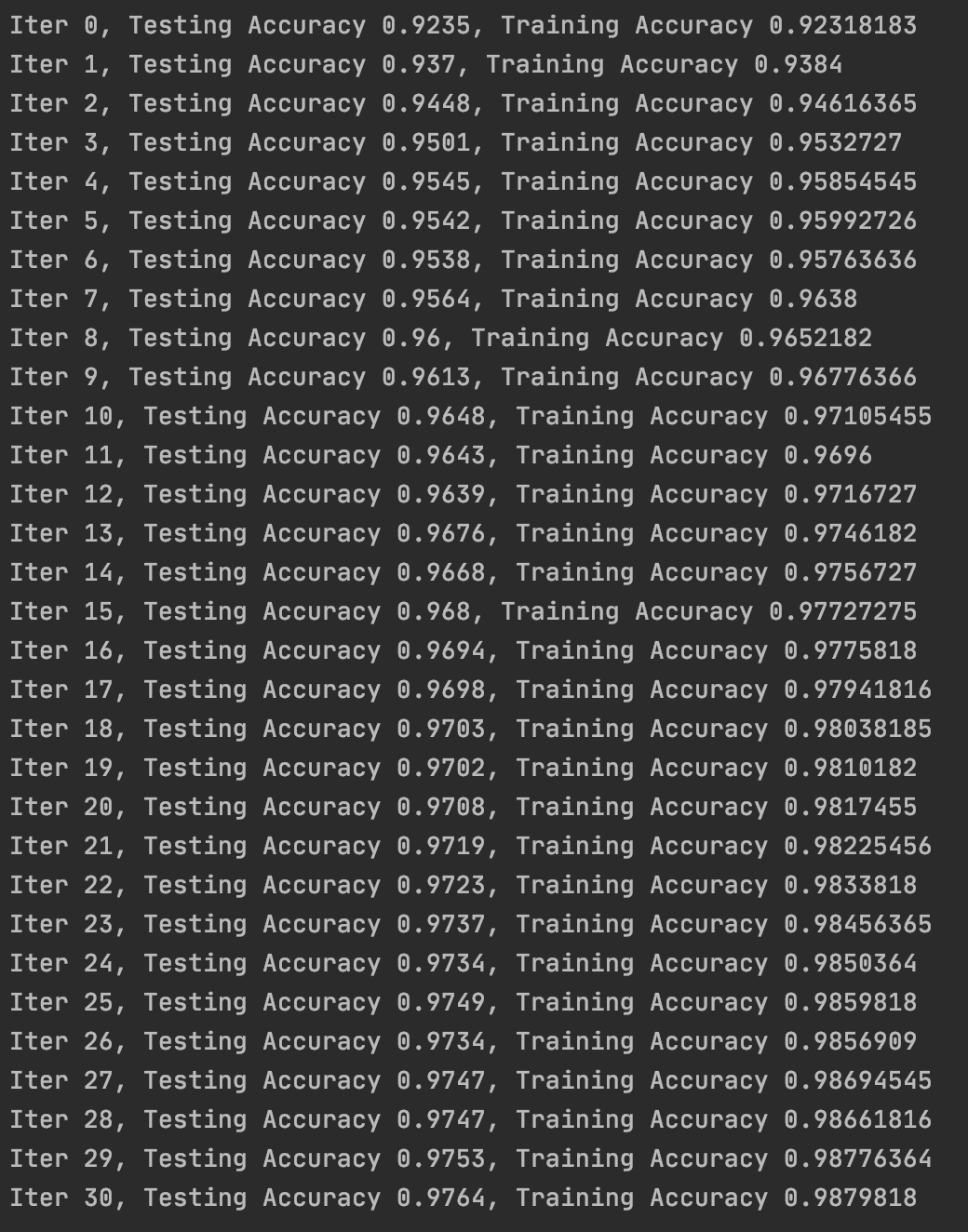# 【Tensorflow基础】第五课：模型优化

## 代价函数，网络结构，优化器，tf.truncated_normal

Posted by x-jeff on May 25, 2020

# 2.修改代价函数

1
loss=tf.reduce_mean(tf.nn.softmax_cross_entropy_with_logits(labels=y,logits=prediction))# 3.修改网络

1. 添加隐藏层。
2. 随机初始化权重。
3. dropout防止过拟合。

1
2
3
4
5
6
7
8
9
10
11
12
13
14
15
16
17
18
19
#第一层
W1=tf.Variable(tf.truncated_normal([784,2000],stddev=0.1))
b1=tf.Variable(tf.zeros()+0.1)
A1=tf.nn.tanh(tf.matmul(x,W1)+b1)
A1_drop=tf.nn.dropout(A1,keep_prob)
#第二层
W2=tf.Variable(tf.truncated_normal([2000,2000],stddev=0.1))
b2=tf.Variable(tf.zeros()+0.1)
A2=tf.nn.tanh(tf.matmul(A1_drop,W2)+b2)
A2_drop=tf.nn.dropout(A2,keep_prob)
#第三层
W3=tf.Variable(tf.truncated_normal([2000,1000],stddev=0.1))
b3=tf.Variable(tf.zeros()+0.1)
A3=tf.nn.tanh(tf.matmul(A2_drop,W3)+b3)
A3_drop=tf.nn.dropout(A3,keep_prob)
#输出层
W4=tf.Variable(tf.truncated_normal([1000,10],stddev=0.1))
b4=tf.Variable(tf.zeros()+0.1)
prediction=tf.nn.softmax(tf.matmul(A3_drop,W4)+b4)


1
2
3
4
5
6
7
8
truncated_normal(
shape,#输出张量围度
mean=0.0,#均值
stddev=1.0,#标准差
dtype=dtypes.float32,#输出类型
seed=None,#随机数种子
name=None#运算名称
)


keep_prob在训练时设为0.7，预测时不能使用dropout，因此预测时keep_drop设为1.0。# 4.修改优化器

1. Adam优化算法tf.train.AdamOptimizer()
2. RMSProp优化算法tf.train.RMSPropOptimizer()
3. Momentum优化算法tf.train.MomentumOptimizer()

1# 5.学习率衰减

1
tf.assign(lr,0.001*(0.95**epoch))# 6.代码地址